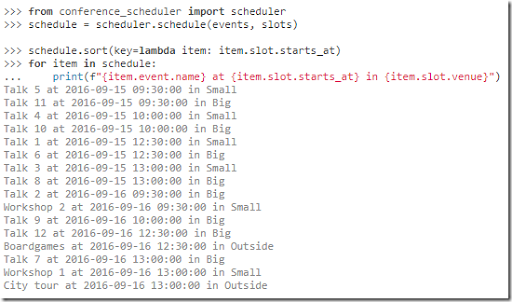## Monday, September 18, 2017

### Conference SchedulerIn [1,2] a Python-based conference scheduler is presented. The problem is as follows:

• There are events (i.e. talks) denoted by $$i$$ and slots denoted by $$j$$
• Some restrictions include:
• Do not schedule talks by the same speaker at the same slot
• Assign events to slots such that one of the following objectives is optimized:
• Minimize total room overflow (that is: demand exceeds capacity)
• Minimize maximum room overflow
• Minimize changes from previous schedule

In  the model is stated as follows:

 \bbox[lightcyan,10px,border:3px solid darkblue]{ \begin{align}\min\>&\sum_{i,j} (d_i-c_j) x_{i,j}&&\text{Sum of room overflow}\\ &\sum_j x_{i,j} =1\>\forall i \\ &\sum_i x_{i,j} \le 1\>\forall j \\ &x_{i,j} = 0\> \forall i,j \in C_S && \text{Unavailability} \\ &x_{i,j} + x_{i’,j’} \le 1\> \forall i,j,i’,j’ \in C_E &&\text{Not at same day}\\ &x_{i,j} \in \{0,1\}\end{align}}

You can choose any single one from the objectives:

 \begin{align}1.&\sum_{i,j} (d_i-c_j) x_{i,j}&&\text{Sum of room overflow}\\2.&\max_{i,j} (d_i-c_j) x_{i,j}&&\text{Max of room overflow}\\3.&\sum_{i,j} \left[(1-x^0_{i,j})x_{i,j}+x^0_{i,j}(1-x_{i,j})\right]&&\text{Difference from a previous solution x^0}\end{align}

The model can be solved with a MIP solver (via the PULP Python package) or using a simulated annealing code.

This is a nice, clean model. Of course there are always things I probably would do differently, largely based on taste.

1. When I did something like this I used a variable $$x_{e,r,t}$$ with $$r,t$$ indicating the room and the time period. In this package $$j$$ captures both $$(r,t)$$. As the package supports events and slots of different length (e.g. 30, 60, 90 minutes) that makes sense.
2. Objective 1 seems to prefer assignments with $$d_i \ll c_j$$. This can be fixed by using: $$\sum_{i,j} \max(d_i-c_j,0) x_{i,j}$$. Of course we can do even better by also penalizing assignments of small events to big rooms, as shown in the rightmost picture:3. It is not always easy to choose between the sum vs the max (objectives 1 and 2). Actually often a combination works very well.
4. Similar for the last objective. In general I combine this with the other objectives, so that we can deviate from an existing schedule if it pays off for another objective.
5. Instead of fixing variables $$x$$ to zero if assignments are forbidden (and leave it to the presolver to get rid of these variables) I like to not even generate these variables. I guess I am old-fashioned.
6. I probably would make the model very elastic: make sure we can always produce a feasible schedule (at the cost of renting very expensive “emergency” rooms). This way we can at least see a solution instead of just a message “infeasible”.
7. The input data in the tutorial seems to repeat much data. E.g. the room capacity is entered for each time slot. It is usually a good idea to “normalize” data: enter the “elementary” data only once.
8. I often prefer to present results in spreadsheet tables, in hopefully a meaningful layout. That seems to work better as a communication tool than a text file:OK, enough of the nitpicking.  In any case, interesting stuff.

##### References
1. A Python tool to assist the task of scheduling a conference, https://github.com/PyconUK/ConferenceScheduler